# Markov Chain Probability

Often during interviews, probability questions are stated that contain a Markov chain. A Markov chain is a sequence of random variables with the property that given the present state, the future states and the past states are independent. In other words, in case the current state is known, the past states do not affect the future. Think for example about a cube. An ant is starting at a specific corner of the cube and it tries to reach the exact opposite corner of the cube by only walking straight to neighbor corners. It takes an ant a second to walk a side. A problem statement – containing a Markov chain – could be to compute the expected amount of time that the ant is walking before it reaches the opposite corner. The ant is moving randomly to one of either three neighbor corners. Past choices do not affect the next choice of the ant, which makes this a Markov chain problem. This section will elaborate on the Markov chain.

In case the problem contains a finite-state Markov chain with n-states, then the Markov chain can be described by an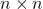transition matrix P, given by Equation 1.

(1)where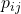is the transition probability from stateto state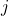. Although a transition matrix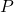already gives an overview of the possible paths, a transition graph makes it even more clear. All the possible and impossible transitions are easy to recognize in a transition graph.

The corresponding transition matrix would be:

(2)In the transition graph, we can see which state is accessible from which state(s). For example, state 4 is not directly accessible from state 1, because one has to apply first for the function before the applicant gets the offer. When two state are mutually accessible, then those state communicate. For example, states 2 and 3 do communicate, as do 1 and 2. State 4 is accessible from state 3, but does not communicate with state 3. A stateis recurrent if for every statethat is accessible from,is also accessible from. A state is called transient when it is not recurrent.

In every problem statement, we are looking for a certain answer. This answer should be the end of the Markov chain, also called the absorbing state. It is impossible to leave this state. In the example with the ant, the absorbing state is when the ant reached the exact opposite corner with respect to the corner from where it started. In the transition graph above, the absorbing state is state number 4. In this case it is assumed that one does not continue with looking for another job after the applicant gets a job offer in trading.

The problem statement could either aim for the probability to reach a specific absorbing state or for the expected number of steps or amount of time before reaching the absorption state. The equation to solve this problem can be derived from the law of total expectation.

Let’s first elaborate on the probability to reach a specific absorption state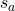. All states are denoted by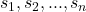for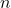-states. The absorption state, and all other states are equal to zero. For every transient statewe have the equation

(3)The equation to solve the number of steps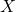before reaching the absorption state is slightly different. Here, the absorption state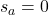. For every transient statewe have the equation

(4)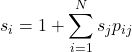These equations can be solved by writing out the equation from the starting point. Note: the ‘1’ in this question stands for each new step that has to be taken to go to the next state. If we would have been talking in terms of time, for example seconds, the ‘1’ in this equation could be replaced by the number of seconds that it takes to reach a next stage.

Example: The ant starts at a specific corner of a cube and tries to reach the exact opposite corner of the cube. It only walks directly to neighbor corners with an equal probability, 1/3. Every time the ant travels to the next corner, a second passes. What is the expected number of seconds that it takes the ant to reach the exact opposite corner?

Solution: First start with numbering all corners to get a clear overview of all states. The ant starts at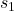and wants to reach.

Now we can start writing out all state equations:

(5)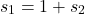(6)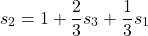(7)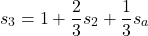is equal to zero as we explained before. We can now substitute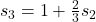in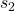. This gives us:

(8)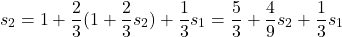(9)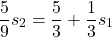(10)Now we can substitutein:

(11)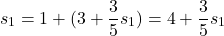(12)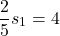(13)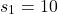In other words, the expected time that it takes the ant to reach the corner in the exact opposite corner is equal to ten seconds. Other problems and solutions can be found in de exercises section.